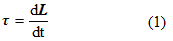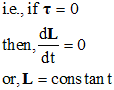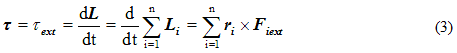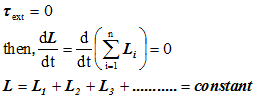# Law of conservation of angular momentum

## Law of conservation of angular momentum

>
• Torque acting on any particle is given byIf external torque acting on any particle is zero then,• Hence in absence of external torque the angular momentum of the particle remains constant or conserved.
• Total torque acting on any system is given by• If total external force acting on any particle system is zero or,• If total external torque acting on any body is zero , then total angular momentum of the body remains constant or conserved.

Question 1 An ice skater spins with arms outstretch at 1.9 rev/s.Her moment of inertia at this time is 1.33 kgm2.She pulls her arms to increase her rate of spin.Her moment of inertia after she pulls her arm is .48kgm2.What is her new rate of spinning
a. 5.26 rev/s
b. 5.2 rev/s
c 4.7 rev/s
d. none of these

Solution 6

Law of conservation of angular momentum

I1ω1=I2ω2
or

1.33(1.9)=.48ω2
or
ω2=5.26 rev/s